• #include&lt;iostream&gt; #include&lt;array&gt; using std::array; template&lt;typename T&gt; void showarray(array&lt;T,10&gt; myarray,int n) { using namespace std;...
#include<iostream>
#include<array>
using  std::array;

template<typename T>
void showarray(array<T,10> myarray,int n)
{
using namespace std;
cout << "TTTTT" << endl;
for (int i = 0; i < n;i++)
{
cout << myarray[i] <<" ";
}
cout << endl;

}

template<typename T>
void showarray(array<T*, 10>  myarray, int n)
{
using namespace std;
cout << "T*T*T*T*T*" << endl;
for (int i = 0; i < n; i++)
{
cout << *myarray[i] << " ";
}
cout << endl;

}

void main()
{

array<int, 10> intarray = { 1, 2, 3, 4, 5,6,7,8,9,10 };
array<int*, 10> pintarray ;
for (int i = 0; i < 10; i++)
{
pintarray[i] = &intarray[i];
}
array<int**, 10> ppintarray;
for (int i = 0; i < 10; i++)
{
ppintarray[i] = &pintarray[i];
}
showarray(intarray, 10); //根据不同参数 选择执行函数
showarray(pintarray, 10);//int*
showarray(ppintarray, 10);//int**

std::cin.get();
}
展开全文• 模板分为模板函数模板类。 如果是交换两个数据，我们会定义对应类型的函数，比如要交换int类型数据，我们会定义int类型swap函数，如果是交换double类型数据，会再定义double类型交换函数。 void Swap(int&...
模板是泛型编程的基础。所谓泛型编程就是编写与类型无关的逻辑代码，是一种复用的方式。模板分为模板函数和模板类。
如果是交换两个数据，我们会定义对应类型的函数，比如要交换int类型数据，我们会定义int类型swap函数，如果是交换double类型数据，会再定义double类型交换函数。
void Swap(int& a, int &b)
{
int c = a;
a = b;
b = c;
}
void Swap(double& a, double &b)
{
double c = a;
a = b;
b = c;
}

这样重复定义函数，代码重复度十分高，但是模板来说不需要用户自己定义多种类型的函数，只需要定义一个模板就可以。模板分为函数模板和类模板。
函数模板 函数模板代表了一个函数家族，该函数模板与类型无关，在使用时被参数化，根据实参类型产生函数的特定类型版本
函数模板格式
template<typename 形参名1,typename 形参名2，typename形参名3>
返回类型  函数名（参数列表）
{…}
模板参数的定义既可以用typename,也可以用class(但是不能用struct),含义是相同的
template<typename T>  //模板参数的定义
void Swap( T& a,  T& b)
{
T c = a;
a = b;
b = c;  //没有实例化不会报错
}
如果函数模板内部出现错误，编译期间将不会报错，因为是在实例化时才检查模板内容,即当用Swap(a,b)会检查模板内容
但是当函数模板头出现问题时，会立即报错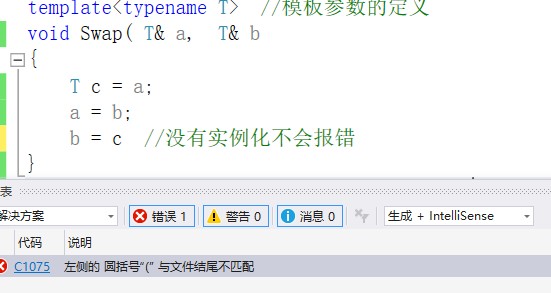int main()
{
int a = 1, b = 2;
double c = 2.2, d = 3.4;
Swap(a, b);
Swap(c, d);
cout << a << endl << b << endl;
cout << c << endl << d << endl;

system("pause");
return 0;
}

汇编看函数模板实例化过程：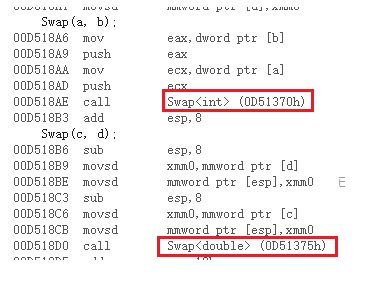模板参数的原理：
模板本身不是类或函数，编译器用模板产生指定的类或函数的特定类型版本，产生模板特定类型的过程称为函数的模板实例化。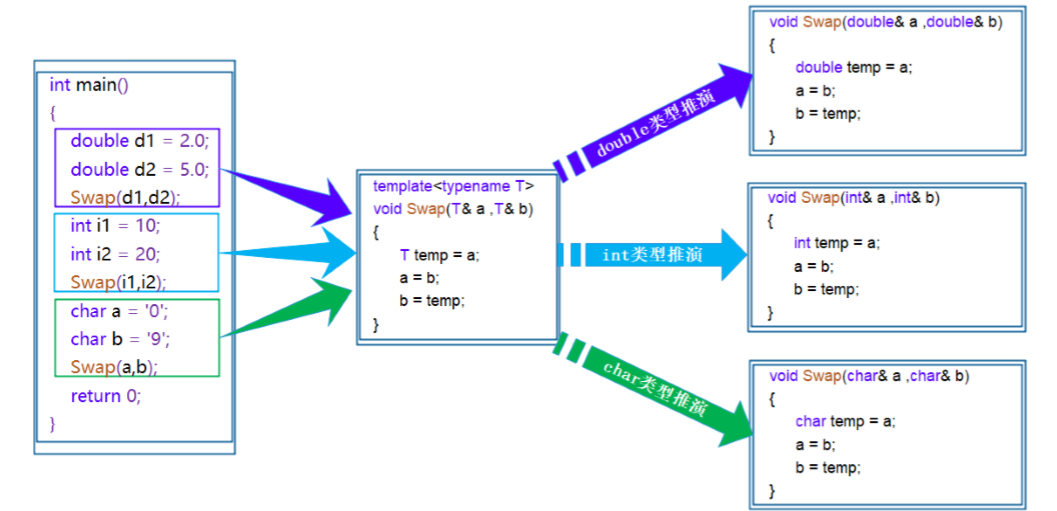在使用函数模板时，编译器需要根据传入的实参类型，推演生成对应类型的函数来使用。
比如int类型使用函数模板时，编译器通过对实参类型的推演，将确定为int类型，最后会生成int类型的数据交换函数
函数模板的实例化 用不同类型的参数使用函数模板时称为函数模板的实例化，模板参数实例化分为隐式实例化和显示实例化
template<typename T>
{
return a + b;
}
int main()
{
int a = 1, b = 2;
double c = 2.2, d = 3.4;
//cout << Add(a, d) << endl;
//在编译期间，当编译器看到该实例化时，需要推演实参类型，通过第一个参数a将T推演为int,
//第二个推演为double,但是，模板参数类型只有一个T，编译器无法确定将T推演为int或者double而出错
//因此需要将类型转换为相同的，可以用户自己强转即隐式类型转换，也可以用显示类型转换
//隐式类型转换
cout << Add(a, b) << endl; //2
cout << Add(a, (int)d) << endl;//4
cout << Add((double)a, d) << endl; //4.4
//显示类型转换:在函数名后加<具体类型>
cout << Add<int>(a, d) << endl; //4
system("pause");
return 0;
}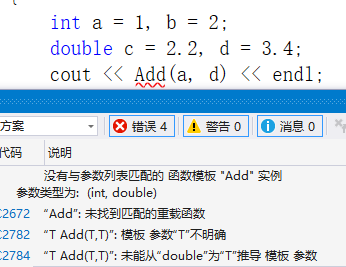函数模板重载
template<typename T>
{
return a + b;
}
{
return a + b;
}
template<typename T1,typename T2>
{
return a + b;
}
int main()
{
int a = 1, b = 2;
double c = 1.2, d = 3.5;
//根据参数找不同模板实例化
system("pause");
return 0;
}



展开全文• //函数模板重载 template <class T>void Swap(T &a, T&b); template <typename T> void Swap(T a[], T b[], int len); void printArray(int arr[],int len); int main() { int m = 10, n =...
#include<iostream>
using namespace std;
//函数模板的重载
template <class T>void Swap(T &a, T&b);
template <typename T> void Swap(T a[], T b[], int len);

void printArray(int arr[],int len);

int main()
{
int m = 10, n = 90;
Swap(m, n);
cout << "m = "<< m << ",n = " << n << endl;

//交换两个数组
int a = { 1, 2, 3, 4, 5 };
int b = { 10, 20, 30, 40, 50};
int len = sizeof(a) / sizeof(int);
Swap(a, b, len);
cout << "数组a：" << endl;
printArray(a,len);
cout << "数组b：" << endl;
printArray(b, len);
return 0;

}

template <class T> void Swap(T &a,T &b)
{
T temp = a;
a = b;
b = temp;
}

template<typename T> void Swap(T a[],T b[],int len)
{
T tmp;
for (int i = 0; i < len;i++){
tmp = a[i];
a[i] = b[i];
b[i] = tmp;
}
}

void printArray(int arr[],int len)
{
for (int i = 0; i < len; i++){
if (i == len-1){
cout << arr[i] << endl;
}
else{
cout << arr[i] << " ";
}
}
}



展开全文c++
• 1.函数模板泛型的实际例子 #include <iostream> using namespace std; template <typename T> void Swap(T &a, T &b) { T temp; temp = a; a = b; b = temp; } int main(int argc, ch....
文章目录1.函数模板泛型的实际例子1.1 隐式推导1.2 显式调用1.3 查看具体调用的是哪一个函数2. 函数模板的重载2.1 函数模板重载和普通函数匹配的规则匹配的规则类模板泛型的实际例子类模板的实际例子总结
1.函数模板泛型的实际例子
1.1 隐式推导
即根据传递的参数来推导出用什么类型来替换T
#include <iostream>

using namespace std;

template <typename T>

void Swap(T &a, T &b)
{
T temp;

temp = a;
a = b;
b = temp;
}
int main(int argc, char *argv[])
{
int a = 2;
int b = 3;

Swap(a, b);

cout << "a=" << a << ", b=" << b << endl;
return 0;
}


运行结果
lydia@lydia:~/sgy/c++_learn$./test a=3, b=2  这个T不是一个具体的数据类型，而是泛指任意的数据类型！ 1.2 显式调用 显式调用int类型的模板 Swap(a,b);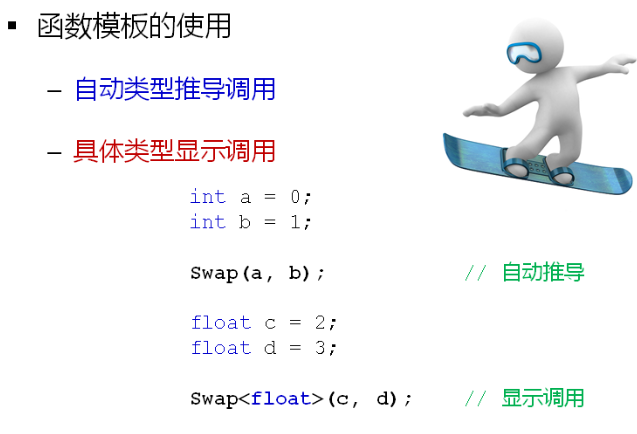1.3 查看具体调用的是哪一个函数 __PRETTY__FUNCTION__  2. 函数模板的重载 函数模板也可以重载！ 下面两个函数是重载函数！ T add(T &a, T &b); T add(const T &a, const T &b);  #include <iostream> using namespace std; template <typename T> T add(T &a, T &b) { cout << __PRETTY_FUNCTION__ << endl; return a+b; } template <typename T> T add(const T &a, const T &b) { cout << __PRETTY_FUNCTION__ << endl; return a+b; } int main() { int a = 1; int b = 2; cout << add(a, b) << endl; return 0; }  执行的结果！ lydia@lydia:~/sgy/c++_learn/template$ ./test
T add(T&, T&) [with T = int]
3
int main()

2.1 函数模板重载和普通函数匹配的规则
这里面有三个函数都匹配，但是优先是调用普通函数！
#include <iostream>

using namespace std;

template <typename T>
{
cout << __PRETTY_FUNCTION__ << endl;
return a+b;
}

template <typename T>
T add(const T &a, const T &b)
{
cout << __PRETTY_FUNCTION__ << endl;
return a+b;
}

{
cout << __PRETTY_FUNCTION__ << endl;
return a+b;
}
int main()
{
int a = 1;
int b = 2;
cout << add(a, b) << endl;
return 0;
}

执行的结果！
lydia@lydia:~/sgy/c++_learn/template$./test int add(int, int) 3  匹配的规则 根据参数转换，列出所有可能匹配的函数 选择最优匹配的函数 如这里2,3函数最优 优先选择普通函数！ 模板之间选择最优！如1,2之间选择 2！ 5. T add(const T &a, const T &b)------->int add(const int &a, const int &b) 6. T add(T &a, T &b)------------>int add(int &a, int &b); 7. int add(int a, int b);  类模板泛型的实际例子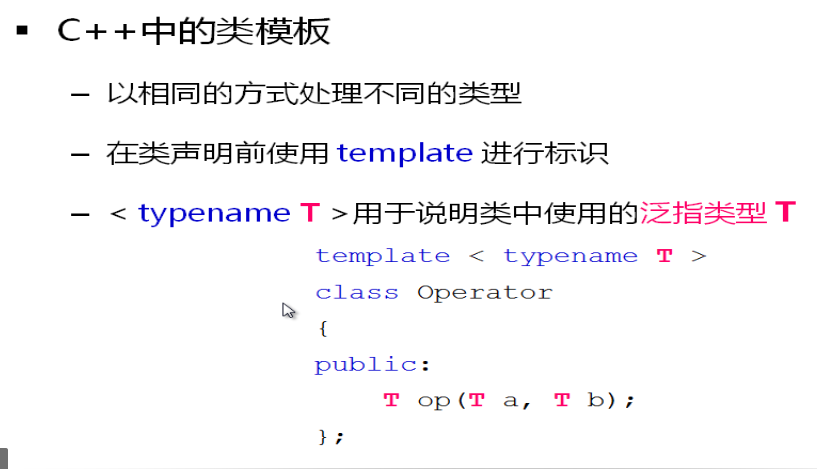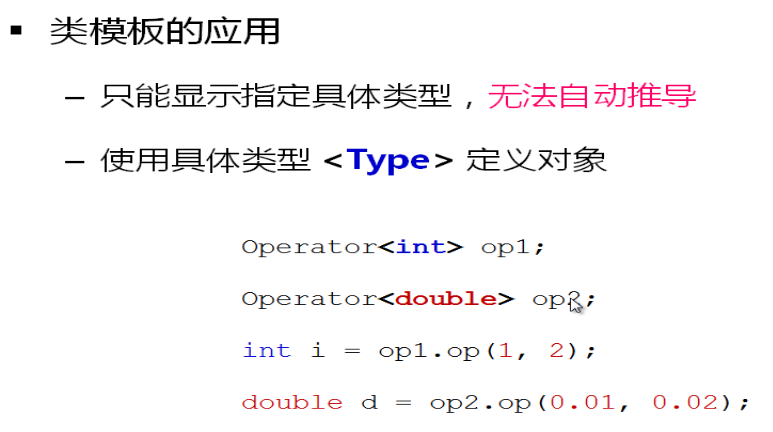类模板的实际例子 #include <iostream> using namespace std; template <typename T> class Op { public: T process(T value) { return value * value; } }; int main(int argc, char *argv[]) { Op<int> opInt; Op<double> opDouble; int a = 2; double d1 = 0.2; cout << "2 * 2=" << opInt.process(a) << endl; cout << "0.2 * 0.2=" << opDouble.process(d1) << endl; return 0; }  执行的结果！ lydia@lydia:~/sgy/c++_learn$ ./test
2 * 2=4
0.2 * 0.2=0.04

总结展开全文• 函数模板可以像普通函数那样重载。 C++ 编译器会从不同的候选中匹配一个并进行调用。 即使不涉及到模板，这种匹配的规则也很复杂，现在还有加上模板一起匹配。 先来个小例子： #include <iostream> // ...
• #include<iostream> using namespace std; template<typename T> void display(T a) { cout<<a<<endl; } template<typename T,class S> void display(T t,S s) ...t&l...vc++6.0
• http://blog.csdn.net/tototuzuoquan/article/details/38626035
• 如果某个成员函数的实现，仅有当前的类型实参不够，则可将该成员函数定义未函数模板，扩展需要的传参类型。 不够有两两层含义： a. 类型实参的量不够； b. 模板函数的传参需要转化。 模板类和模板函数的差别： a...
• //函数模板重载 #include<iostream> using namespace std; /* 函数模板会进行严格的类型匹配，模板类型不提供隐式类型转化 普通函数能够进行自动类型转换 */ /* 函数模板重载四大规则 1 函数模板...
• 1、重载：函数名相同，但函数的...（2）若普通函数没有，则在函数模板中查找，将模板实例化，若和调用的函数匹配，则调用该函数模板； （3）若经过上述两步还是未能找到，则可以通过类型转换转换进行参数匹配的函数重
• 可以用相同的函数名重载函数模板 编译器在处理程序中的函数调用时 必须能够知道哪一个模板或普通函数是最适合调用的函数 //: C05:MinTest.cpp // From "Thinking in C++, Volume 2", by Bruce Eckel &...
• http://blog.csdn.net/tototuzuoquan/article/details/38626035python java matlab opencv c++
• ﻿﻿ ...//函数模板可以对类型进行优化重载，根据类型会覆盖 //如果仍然要使用模板函数，需要实例化   templateclass T> T add(T a, T b) {   std::cout "T add " <<
• #include <iostream> #define WAY 1 template <...是使用了Screen的默认构造函数吗？可是默认构造函数不能初始化成员，为什么在输出screen的时候可以输出我输入的的height和length呢
• 1、函数重载（多态） 2、函数模板及其重载 3、完全匹配与最佳匹配C++ 多态 函数
• 直接上代码   #include&lt;iostream&gt; using namespace std; template &lt;class T&gt; void print(T a) { cout &lt;&lt; "first\n"&lt;&lt;a &...{
• 模板一： template<typename T> string debug_rep(const T &t); 模板二： template<typename T> string debug_rep(T *p)； 使用： string s("hi"); const string *sp=&s; 方式一：debug_rep(&s)； 方式二：...函数参数 引用 指针
• 为什么要用函数模板重载呢？有的时候并非所有的类型都是用相同的算法，为了满足这种需求可以重载常规函数的定义那样重载模板。 下面是一个简单的例子：c++
• 函数重载是C++中实现静态多态的重要机制，在定义函数重载时，特别是在有函数模板加入时，更需要小心定义参数，防止歧义的出现 举例来说，有下面两个重载函数，用于执行数据库查询并返回查询结果，分别对应到查...C++
• #include #include using std::strcmp; using std::cout; using std::endl; templateconst T & min(const T & a,const T & b){ cout模板被调用！"; return (a)?a:b; } const char * min(const char * a,constC++
• 支持泛型编程是c++非常强大的一个特性，我们可以通过定义一个模板函数和模板类，...首先我们还是以一个例子来开始，我定义了一个如下的函数模板用来实现，两个对象的比较功能： 点击(此处)折叠或打开 template
• template<class T> void fun(const T& a,const T& b) ...如果都要进行转换,那么普通函数应该要优先于模板函数才对. 但是为什么结果是调用模板函数呢?难道模板函数不需要类型转换吗???
• 一、引子 考虑求两数较大值函数max(a,b) 对于a，b的不同类型，都有相同的处理形式： return a &lt; b ? b : a; ...（1）宏替换 #define max(a,b) ((a)&...（3）使用函数模板 二、模板 模板是...
• 所谓的函数模板重载是指，普通函数的版本，函数模板的版本和函数模板特例化的版本可以共存，例如： //普通函数版本 bool Compare(char* a, char* b) { cout << "普通函数版本" << endl; return ...模板
• C++学习笔记函数重载 函数重载 C语言规定在同一作用域（例如同一文件模块中）中不能有同名的函数。 而C++允许在同一作用域中用同一函数名定义多个函数，这些函数的参数个数和参数的类型不相同，这些同名的函数...C++
• 函数模板与函数重载 一，什么是函数重载 1，在理解什么是函数重载之前，先理解一下函数符号生成规则，在C语言中，函数符号的生成只与函数名相关，这个是什么意思呢？如下代码： #include<stdio.h> int sum...c++...